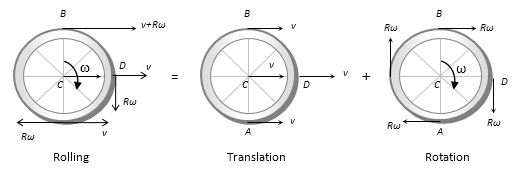Question

# Rolling motion is

Open in App
Solution

## Rolling motion: It is the motion in which the mix of rotation and translation has occurred.A diagram to represent the rolling motion:The formula of rolling motion:$K=\frac{1}{2}m{{v}^{2}}_{cm}+\frac{1}{2}I{\omega }^{2}$where $K$ is the total kinetic energy,$m$ is the mass of the body,${v}_{cm}$ is the velocity of the center of mass of the body,$I$ is the moment of inertia of the body,$\omega$ is the angular velocity of the body.Suggest Corrections1Similar questionsExplore more